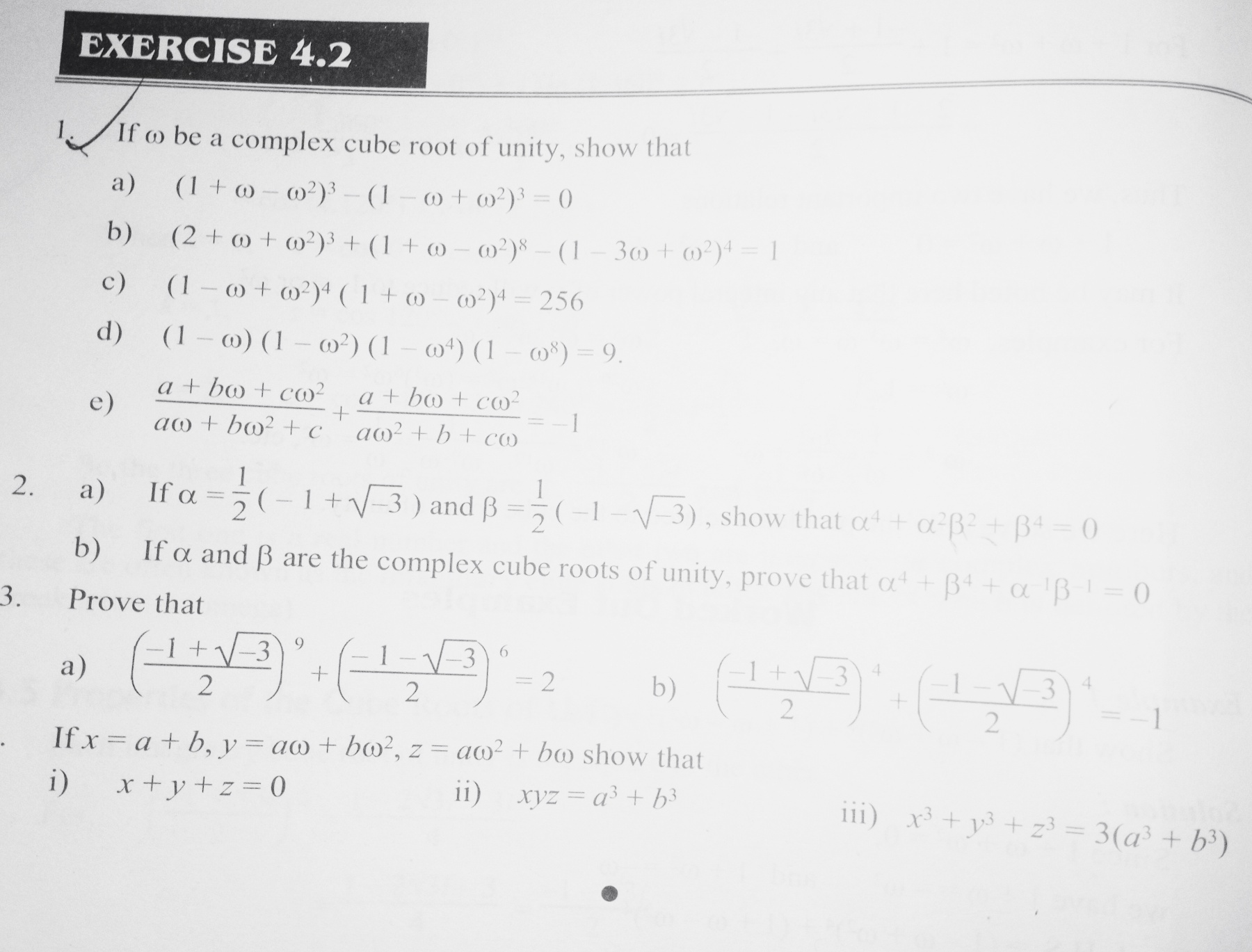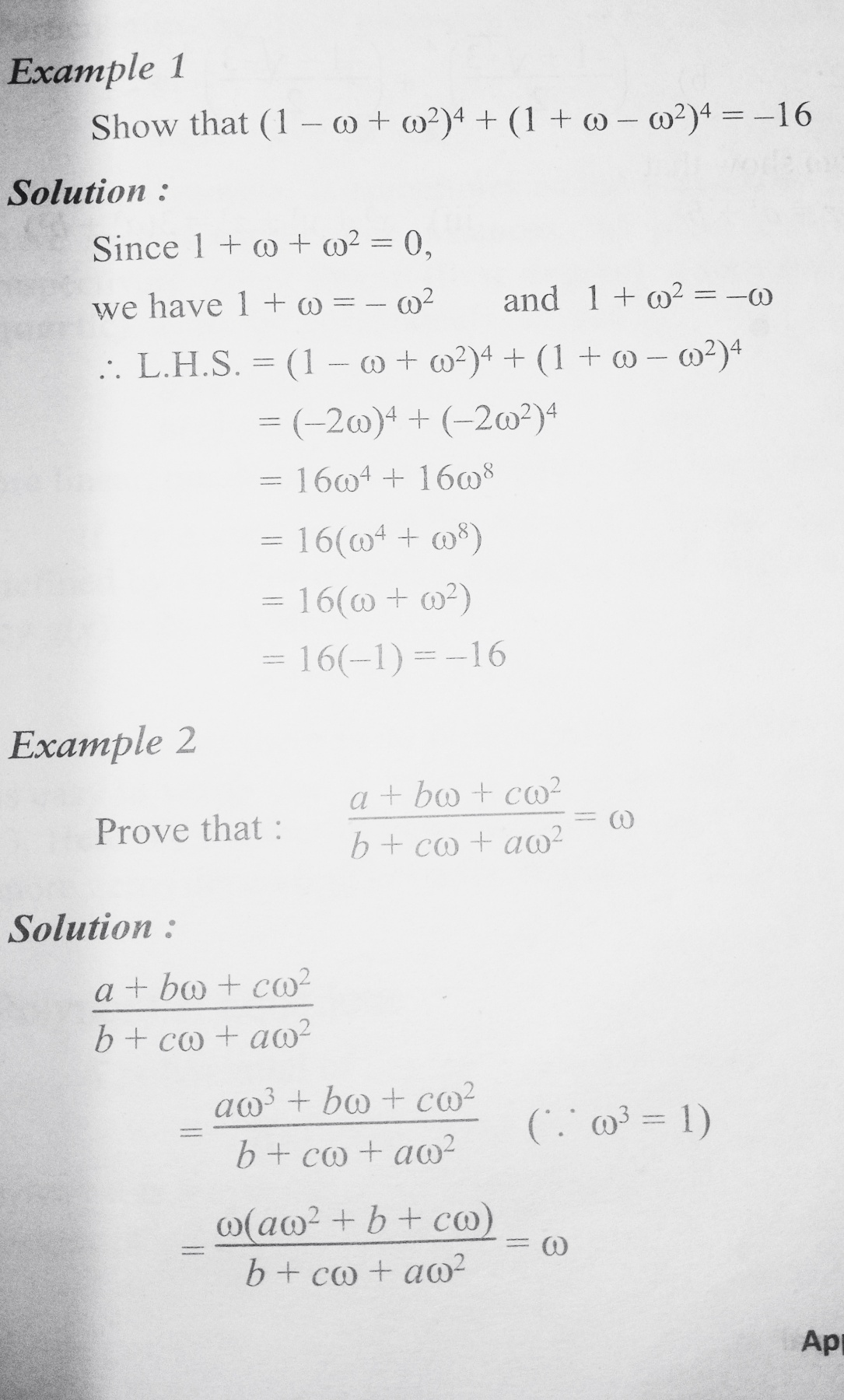# Complex Number - Exercise 4.2 : Class 12 Math

Complete Exercise 4.2 Complex Number. Exercise 4.2: Cube Roots of Unity and its Properties Complete Question Answer Solution, Class 12 Mathematics.

## Chapter 4: Complex Number.

### Exercise: 4.2

Complete Exercise of Complex Number - Exercise 4.2 : Class 12 Mathematics 2080 NEB.

Exercise 4.2 is about: The Cube Roots of Unity and Properties of the Cube Roots of Unity.

#### Complex Number - Exercise 4.1 Questions#### Complex Number - Exercise 4.2 Important Practice Question AnswerGetting Info...